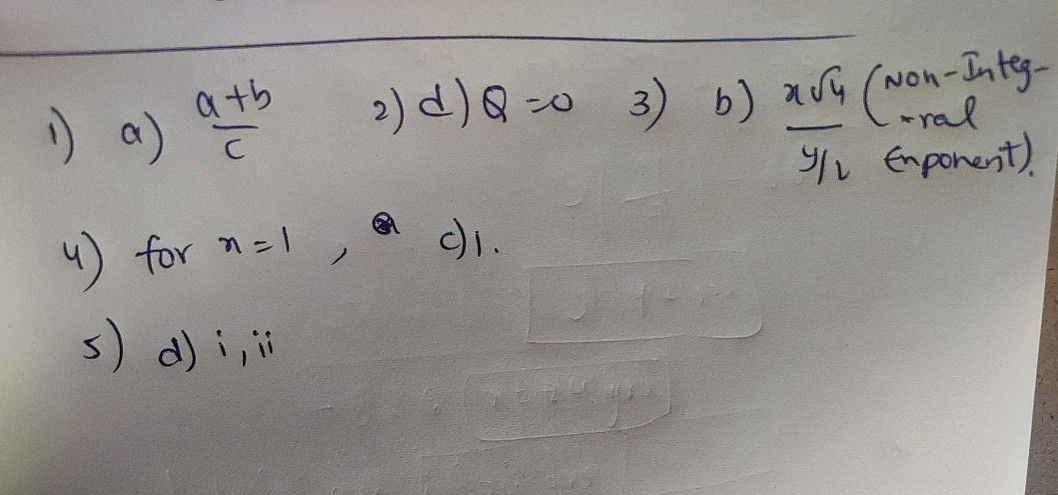Symbol
Problem$DIREc$ $TON$ Answer the $folowing$ questions. $choose$ the $le++er$ of the $correc1$ $answer$ 1. Which of the following expressions is a rational algebraic expression? a. a+C b b. $\dfrac {a^{-3}+b} {c}$ $c.\dfrac {a+b} {c^{\dfrac {1} {2}}}$ d. C a-+1 b $2.1\dfrac {P} {Q}isa$ $a.Q>0$ rational algebraic expression, which of the following conditions is $nco\pi ect7$ $b.Q≠0$ c. Q< 0 $d.Q=0$ 3. Which of the following expressions is NOT a rational algebraic expressions? $c\dfrac {3x^{\dfrac {1} {2}}} {y^{2}+2y+1}$ $d.x^{2}+4x+5$ $a.\dfrac {x+y} {2}$ $b.\dfrac {x\sqrt{4} } {\dfrac {y} {2}}$ 4. What value of x will make the expression x5 -1 meaningless? a. -1 b. 0 C. 1 d. 2 5. Which of the following describes a rational algebraic expression? i. both the numerator and denominator are polynomials ii. no $n0n-intθgra$ exponent iii. the denominator is zero a. i only b. i,ii and ii c. ii and i d. $iandi$